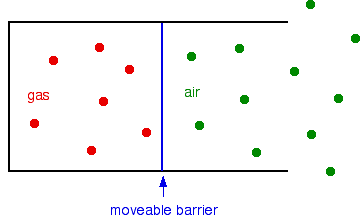# Charles's Law (Law of Volumes)

•• Contributed by Jim Clark
• Former Head of Chemistry and Head of Science at Truro School in Cornwall

Charles' Law

For a fixed mass of gas at constant pressure, the volume is directly proportional to temperature (in Kelvin). This is mathematically

$V = constant x T$

That means, for example, that if you double the temperature from, say to 300 K to 600 K, at constant pressure, the volume of a fixed mass of the gas will double as well. You can express this mathematically as

Is this consistent with pV = nRT ?

• You have a fixed mass of gas, so n (the number of moles) is constant.
• R is the gas constant.
• Charles' Law demands that pressure is constant as well.

If you rearrange the pV = nRT equation by dividing both sides by p, you will get

V = nR/p x T

But everything in the nR/p part of this is constant. That means that V = constant x T, which is Charles' Law.

### Simple Explanation

Suppose you have a fixed mass of gas in a container with a moveable barrier - something like a gas syringe, for example. The barrier can move without any sort of resistance.The barrier will settle so that the pressure inside and outside is identical. Now suppose you heat the gas, but not the air outside. The gas molecules will now be moving faster, and so will hit the barrier more frequently, and harder. Meanwhile, the air molecules on the outside are hitting it exactly as before.

Obviously, the barrier will be forced to the right, and the volume of the gas will increase. That will go on until the pressure inside and outside is the same. In other words, the pressure of the gas will be back to the same as the air again.

So we have fulfilled what Charles' Law says. We have a fixed mass of gas (nothing has been added, and nothing has escaped). The pressure is the same before and after (in each case, the same as the external air pressure). And the volume increases when you increase the temperature of the gas. What we have not shown, of course, is that there is a "directly proportional" relationship.

## Contributors

Jim Clark (Chemguide.co.uk)